Some feedforward neural networks using Keras

In a previous article I explained the way I see neural networks and gave some basic examples. Personally I believe in ‘simple examples’ as a way to comprehend crucial principles and this article continues in this fashion. By looking at a single cell the activation functions are highlighted and it’s shown that picking the most appropriate one can be done using grid-search. Along the way you can see that simple feedforward networks are a way to dissipate noise and that neural networks are really just functions.

Like the previous article, the examples are on top of the Keras framework but you can recreate all of this in TensorFlow, Caffe or any other neural framework.

Feedforward networks are fairly easy but can nevertheless produce great results. One would sometimes forget, considering what the internet is buzzing about, that not everything needs to be casted in convolutional and/or recurrent topologies.

None of the examples require GPU or datacenters, the synthetic or artificial data is designed to highlight a particular aspect and not a real-world case.

Counting from 0 to 9

Let’s start with learning a network to count from 0 to 9. The code predicts the next number for a given sequence of the previous numbers. The number 9 is followed by 0 in cycles.

import numpy as np
from keras.datasets import imdb
from keras.models import Sequential
from keras.layers import Dense

from keras.preprocessing import sequence
from keras.utils import np_utils

base_series = [0,1,2,3,4,5,6,7,8,9]
series = base_series*10
seq_length = len(base_series)
X = []
Y = []
def unit(index): return [1.0 if i == index else 0.0 for i in range(seq_length)]
# make buckets
for i in range(0, len(series) - seq_length, 1):
X.append(series[i:i + seq_length])
Y.append(unit(np.mod(i, seq_length)))
X = np.array(X)
Y = np.array(Y)

model = Sequential()

# try alternatives if you wish

model.compile(loss='mean_absolute_error', optimizer='rmsprop', metrics=['accuracy'])
model.fit(X, Y, nb_epoch=350, verbose=0)
scores = model.evaluate(X, Y, verbose=0)
print("Model Accuracy: %.2f%%" % (scores*100))
Model Accuracy: 100.00%
Note that the data is partitioned in buckets so the to-be-predicted number is not based on a single digit but on a bucket of digits. When data has some time-like ordering one typically uses networks with memories aka recurrence but this bucket approach works just as well in simple situations (i.e. no variations and few features). You should try to make the same prediction network with only a single number. The bucket approach is useful as a preparation for recurrent networks where one typically has this step-backward situation.

Bit shift operator

Like the counting example we take some binary buckets and shift the 1-bits to the right.

import numpy as np
from keras.datasets import imdb
from keras.models import Sequential
from keras.layers import Dense
from keras.layers import LSTM
from keras.layers.embeddings import Embedding
from keras.preprocessing import sequence
from keras.utils import np_utils

X = []
Y = []
train_size = 50
seq_length = 5
def unit(index): return [1.0 if i == index else 0.0 for i in range(seq_length)]
for i in range(train_size):
X.append(unit(np.mod(i, seq_length)) )
Y.append(unit(np.mod(i+1, seq_length)))
X = np.array(X)
Y = np.array(Y)
#print(X.shape, Y.shape)

model = Sequential()

model.compile(loss='mean_absolute_error', optimizer='rmsprop', metrics=['accuracy'])
model.fit(X, Y, nb_epoch=350, verbose=0)
scores = model.evaluate(X, Y, verbose=0)
print("Model accuracy: %.2f%%" % (scores*100))
print("Model loss: %.2f%%" % (scores*100))
# you can see what the network does to the whole training data by means of
# model.predict(X)
# to see the output of a single vector you can use
model.predict(np.array([[0,1,0,0,0]]))
(50, 5) (50, 5)
Model accuracy: 100.00%
Model loss: 0.06%
array([[  3.66928839e-06,   3.68566514e-04,   9.99535322e-01,
9.16142744e-05,   8.26911730e-07]])
The output is not precise but you can truncate it and plot it to see it more clearly.
import matplotlib.pyplot as plt
import numpy as np
%matplotlib inline
s = np.array([[0,1,0,0,0]])
plt.imshow(np.concatenate( (s, model.predict(s)) ), interpolation='nearest', cmap=plt.cm.Greys)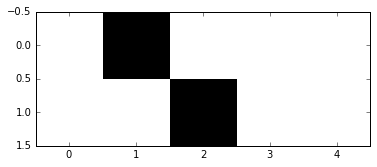Using the score you can see that all solutions give accuracy 100% but the loss differs:
single: 20.62%
one extra: 4.05%
two extra: 5.82%
one (20): 0.24%
two (20): 0.00%

So, you don’t need to increase complexity in order to achieve accuracy but the signal will be more sharp if you do.

The truncation to integers can also be achieved by means of custom layers. Below you can find the Round layer which does precisely this.

Neurons as functions

A single neuron, node or cell is just a function and if you play a bit with the API you can also visualize the various activation functions.
Let’s explicitly assign weights to a single cell thus preventing this to affect the output:

we = [np.array([[0.8]]), np.array([0.])]
model = Sequential()
model.summary()
model.layers.get_weights()
____________________________________________________________________________________________________
Layer (type)                     Output Shape          Param #     Connected to
====================================================================================================
dense_6 (Dense)                  (None, 1)             2           dense_input_4
====================================================================================================
Total params: 2
____________________________________________________________________________________________________
[array([[ 0.80000001]], dtype=float32), array([ 0.], dtype=float32)]

Note that if no activation is specified it will default to linear and that compiling a network will typically assign random weights to the nodes. Details of how the activations are effectively implemeted (really straightforward) can be found here but you can also easily plot the activation functions by using the single cell as a functions:

we = [np.array([[2.]]), np.array([0.])]
def pred(name):
model = Sequential()
return model.predict(np.array([[i] for i in np.arange(-2,2,.1)]))

f, ar = plt.subplots(2, 2, sharey=True)
plt.ylim(-.1,1.1)
ar[0,0].plot(pred("hard_sigmoid"))
ar[0,0].set_title('hard_sigmoid')
ar[0,1].plot(pred("relu"))
ar[0,1].set_title('relu')
ar[1,0].plot(pred("sigmoid"))
ar[1,0].set_title('sigmoid')
ar[1,1].plot(pred("tanh"))
ar[1,1].set_title('tanh')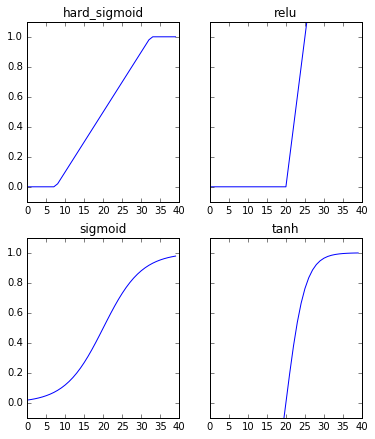If you want a custom activation function you can simply plug in your own function instead of a name (string). Whether something like the sine below makes sense is of course another matter, but you can indeed use anything you like:

def custom(x):
return np.sin(x)**4
we = [np.array([[2.]]), np.array([0.])]
model = Sequential()
pred = model.predict(np.array([[i] for i in np.arange(-2,2,.1)]))
plt.ylim(-.1,1.1)
plt.plot(pred)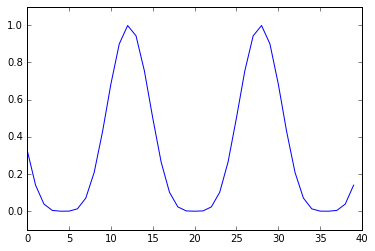Note that activation can be added separately to the model like so

from keras.layers.core import Activation
model = Sequential()
pred = model.predict(np.array([[i] for i in np.arange(-2,2,.1)]))

Finally, there are also advanced activation functions in Keras which are there for specific tasks. Though you can use them like any other activation function, they work well for image-oriented learning. For instance, the parametric rectified linear unit or PReLu function was invented to surpass human-level performance on ImageNet classification.

Picking the most appropriate activation functions

The activation functions seem to be only slightly different but they actually do make a big difference. In the example below we have some artificial data consisting of a line with a bump in the middle together with some noise and try to make the neural network learn the shape of the curve. You can see from the plot below that relu does a much lesser job than the hyperbolic tangent activation.

import matplotlib.pyplot as plt
import numpy as np
%matplotlib inline
from keras.models import Sequential
from keras.layers import Dense
from keras.preprocessing import sequence
from keras.utils import np_utils
from keras.optimizers import *
def shakenGauss(x): return x + 5*np.exp(-(x+2)**2)+0.1*np.random.randn()
shakenGauss = np.vectorize(shakenGauss)

X = np.arange(-7, 7, 0.005)
Y = shakenGauss(X)
plt.plot(X,Y)
# try to use other optimizers to see what it gives
# here the stochastic gradient descent
#https://github.com/fchollet/keras/blob/f127b2f81d5d71fa9ab938ba6f42866d31864259/keras/optimizers.py#L114
# lr: learning rate or how fast the minimum is reached
opt = SGD(lr=0.001)

def fit(activationName):
model = Sequential()
model.compile(loss='mean_absolute_error', optimizer=opt, metrics=['accuracy'])
model.fit(X, Y, nb_epoch=800, verbose=0)
return model

model1 = fit("tanh")
pred1 = model1.predict(X)
# metrics from the evaluate process can be fetched from model1.metrics_names
print("\ntanh loss: %s "%model1.evaluate(X,Y))
plt.plot(X, pred1, color="orange")

model2 = fit("relu")
pred2 = model2.predict(X)
print("\nrelu loss: %s "%model2.evaluate(X,Y))
plt.plot(X, pred2, color="red")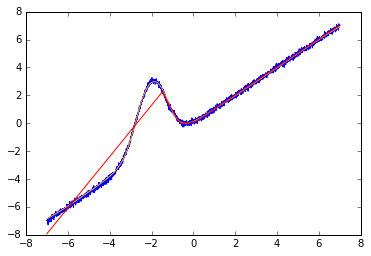How can one optimize this and pick up the most appropriate activation? You can loop over the various activations or use the sklearn wrapper for Keras which allows you to use Keras networks as machine learning models in sklearn.

import numpy
from sklearn.grid_search import GridSearchCV
from keras.models import Sequential
from keras.layers import Dense
from keras.wrappers.scikit_learn import KerasClassifier
from sklearn.metrics import make_scorer
def shakenGauss(x): return x + 5*np.exp(-(x+2)**2)+0.1*np.random.randn()
shakenGauss = np.vectorize(shakenGauss)

X = np.arange(-5, 5, 0.05)
Y = shakenGauss(X)
def create_model(activationName):
model = Sequential()
model.fit(X, Y, nb_epoch=100, verbose=0)
return model
def overall_average_score(actual,prediction):
return np.average(np.abs(actual - prediction))

model = KerasClassifier(build_fn=create_model, nb_epoch=100, batch_size=10, verbose=0)
activationNames = ['softmax', 'softplus', 'softsign', 'relu', 'tanh', 'sigmoid', 'hard_sigmoid', 'linear']
param_grid = dict(activationName = activationNames)
grid_scorer = make_scorer(overall_average_score, greater_is_better=False)
grid = GridSearchCV(estimator = model, param_grid = param_grid, n_jobs=1, scoring=grid_scorer)
grid_result = grid.fit(X, Y)

print("Best activation is '%s'." % grid_result.best_params_["activationName"])
Best activation is 'softsign'.

The scores can be seen from grid_scores_:

grid.grid_scores_

[mean: -2.11227, std: 1.11519, params: {'activationName': 'softmax'},
mean: -2.34436, std: 1.16588, params: {'activationName': 'softplus'},
mean: -1.97574, std: 0.97005, params: {'activationName': 'softsign'},
mean: -1.97574, std: 0.97005, params: {'activationName': 'relu'},
mean: -2.11227, std: 1.11519, params: {'activationName': 'tanh'},
mean: -2.00716, std: 0.92753, params: {'activationName': 'sigmoid'},
mean: -2.01729, std: 0.91384, params: {'activationName': 'hard_sigmoid'},
mean: -2.01729, std: 0.91384, params: {'activationName': 'linear'}]
You can further refine the network with grid-searching the appropriate optimizer, loss function and pretty much every parameter (including the weights). Ain’t it wonderful you can combine Keras and Scikit-learn? Jason Brownlee has a great blog post on how to do all of this.

The neural network (especially the low-loss ones) approximates the syntehtic function quite well and can see through the super-imposed noise. One could of course filter out the noise in other ways (chi-square or moving averages) but the fact that the network does this without explicitly encoding it is a nice feature on its own.

Cellular automata

Cellular automata are in a way primitive neural networks in the sense that they encapsulate state machines which can be found inside e.g. LSTM nodes. From another angle, a cellular automata is just a (discrete) function and like any other function can be mimiced or approximated by neural nets. The rule 30 used below is a world on its own and one could probably find interesting morphisms (same category?) between the world of automata and the world or neural networks.

# this outputs a piece of automata
def ca_data(rulenum:int = 30, height:int = 50, width:int = 20, dorandom:bool = True ):
if dorandom:
first_row = [np.random.randint(2) for i in range(width)]
else:
first_row = *width
first_row[int(width/2)] = 1
results = [first_row]
rule = [int((30/pow(2,i)) % 2) for i in range(8)]

for i in range(height-1):
data = results[-1]
new = [rule[4*data[(j-1)%width]+2*data[j]+data[(j+1)%width]] for j in range(width)]
results.append(new)
return results

import matplotlib.pyplot as plt
import numpy as np
%matplotlib inline

plt.imshow(ca_data(), interpolation='nearest', cmap=plt.cm.Greys)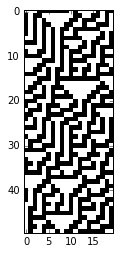Let’s try to use the data to train a dense network. Note that we define a custom layer to output bits and that it’s really easy to add your own modules or layers. Like above, there are other ways to truncate data but this shows how you can plug into the API.
import numpy as np
from keras.datasets import imdb
from keras.models import Sequential
from keras.layers import Dense
from keras.layers import LSTM
from keras.layers.embeddings import Embedding
from keras.preprocessing import sequence
from keras.utils import np_utils
from keras.callbacks import EarlyStopping
from keras.layers.core import Dense, Activation
from keras.models import Sequential
from keras.optimizers import SGD
from keras.layers import Layer
import keras.backend as K

world_size = 20
data = ca_data(30, 1000, world_size, True)
X_train = np.array(data[:-1])
y_train = np.array(data[1:])
test_data = ca_data(30, 100, world_size, True)
X_test = np.array(test_data[:-1])
y_test = np.array(test_data[1:])

# custom Keras layer to truncate floats to bits
class Round(Layer):
def get_output_shape_for(self, input_shape):
return input_shape
return K.round(x)

def build_and_train_mlp_network(X_train, y_train, X_test, y_test):

nb_epoch = 600
batch_size = 10

model = Sequential()

model.fit(X_train,
y_train,
batch_size=batch_size,
nb_epoch=nb_epoch,
verbose=0)
return model

model = build_and_train_mlp_network(X_train, y_train, X_test, y_test)
#np.sum(np.abs(model.predict(X_test) - y_test))
plt.imshow(np.abs(model.predict(X_test) - y_test), interpolation='nearest', cmap=plt.cm.prism)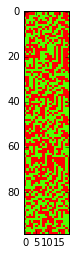It’s clear that this approach is not successful. One way to proceed would be to engage recurrent or convolutional networks. The other is to model the actual rule and not the instances produced by the rule.
The following is a straighforward function prediction model with 100% accuracy.

np.random.seed(233)
ruleNumber = 30
rule = [int((ruleNumber/pow(2,i)) % 2) for i in range(8)]
X = []
Y = []
train_size = 400
X = np.random.randint(0,8, train_size)
Y = [rule[i] for i in X]
model = Sequential()
model.compile(loss='binary_crossentropy', optimizer='rmsprop', metrics=['accuracy'])
model.fit(X, Y, nb_epoch=500, verbose=0)
scores = model.evaluate(X, Y, verbose=0)
print("Model accuracy: %.2f%%" % (scores*100))
print("Model loss: %.2f%%" % (scores*100))
#model.predict(X)
Model accuracy: 100.00%
Model loss: 2.30%

Reuters classification

In this last example we pick up Reuters data which has been preprocessed (as part of the Keras framework). The original data consists of paragraphs but the words have already been embedded (mapped to vectors) and you can extract immediately training and test data.

A word about dropout. This is a way to regularize networks and to suppress overfitting. It effectively switches off some of the neurons (in a random fashion) so that feedback does not affect all of the neurons all the time. Typically you will see that a dropout of half of the nodes is a common approach. Like everything else, some experimentation reveals what works best with the data and what you aim for.

from __future__ import print_function
import numpy as np
np.random.seed(1337)

from keras.datasets import reuters
from keras.models import Sequential
from keras.layers import Dense, Dropout, Activation
from keras.utils import np_utils
from keras.preprocessing.text import Tokenizer

max_words = 1000
batch_size = 100
nb_epoch = 200

(X_train, y_train), (X_test, y_test) = reuters.load_data(nb_words=max_words, test_split=0.2)

nb_classes = np.max(y_train)+1

tokenizer = Tokenizer(nb_words=max_words)
X_train = tokenizer.sequences_to_matrix(X_train, mode='binary')
X_test = tokenizer.sequences_to_matrix(X_test, mode='binary')

Y_train = np_utils.to_categorical(y_train, nb_classes)
Y_test = np_utils.to_categorical(y_test, nb_classes)

model = Sequential()

history = model.fit(X_train, Y_train, nb_epoch=nb_epoch, batch_size=batch_size, verbose=0, validation_split=0.1)
score = model.evaluate(X_test, Y_test, batch_size=batch_size, verbose=1)
print("\n\nModel accuracy: %.2f%%" % (score*100))
print("Model loss: %.2f%%" % (score*100))
Model accuracy: 78.58%
Model loss: 169.86%
This gives around 80% accuracy in very little time (couple of minutes). If you try the same dataset with XGBoost it will take quite a long time (around 10 minutes) for the same 80% accuracy:
import numpy
import xgboost # might require 'pip install xgboost'
from sklearn import cross_validation
from sklearn.metrics import accuracy_score
(X_train, y_train), (X_test, y_test) = reuters.load_data(nb_words=max_words, test_split=0.2)
nb_classes = np.max(y_train)+1

tokenizer = Tokenizer(nb_words=max_words)
X_train = tokenizer.sequences_to_matrix(X_train, mode='binary')
X_test = tokenizer.sequences_to_matrix(X_test, mode='binary')

Y_train = np_utils.to_categorical(y_train, nb_classes)
Y_test = np_utils.to_categorical(y_test, nb_classes)

model = xgboost.XGBClassifier()
model.fit(X_train, y_train)

y_pred = model.predict(X_test)
predictions = [round(value) for value in y_pred]

accuracy = accuracy_score(y_test, predictions)
print("Accuracy: %.2f%%" % (accuracy * 100.0))

For sure one can tune both approaches but it shows that neural nets are not necessarily data and processing hungry in all cases and that neural networks are easy to play with. At least, if you use a framework like Keras.